# Number of Weeks Between Dates in Excel & Google Sheets

This tutorial will demonstrate how to calculate the number of weeks between two dates in Excel and Google Sheets.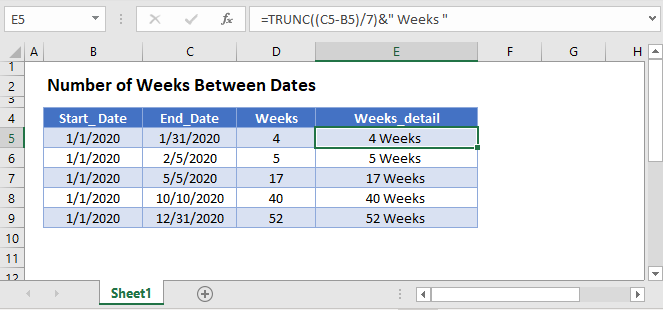## Weeks Between Dates

To calculate the number of weeks between two dates, first calculate the number of days between the dates. We will do this by subtracting the dates:

``=C3-B3``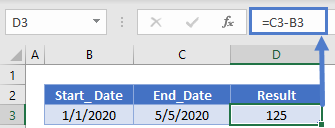Next we will divide the number of days by 7 to calculate the number of weeks:

``=(C3-B3)/7``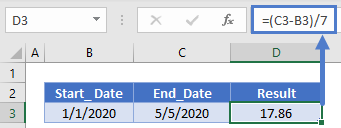We can calculate the number of full weeks with the TRUNC or INT Functions:

``=TRUNC((C3-B3)/7)``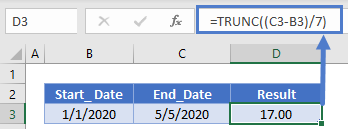Or you can use the ROUNDUP Function so that a partial week is counted as a full week:

``=ROUNDUP((C3-B3)/7,0)``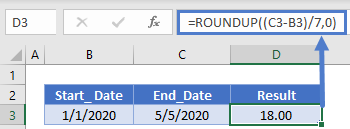## Weeks Between Dates in Google Sheets

All of the above examples work exactly the same in Google Sheets as in Excel.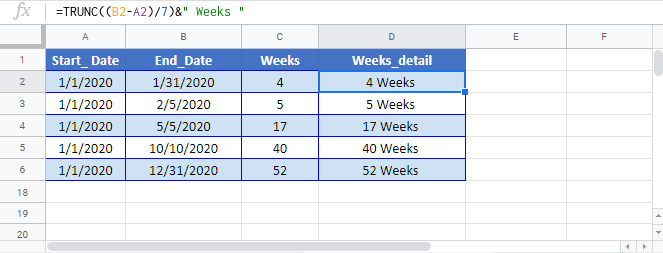### Excel Practice Worksheet

Practice Excel functions and formulas with our 100% free practice worksheets!